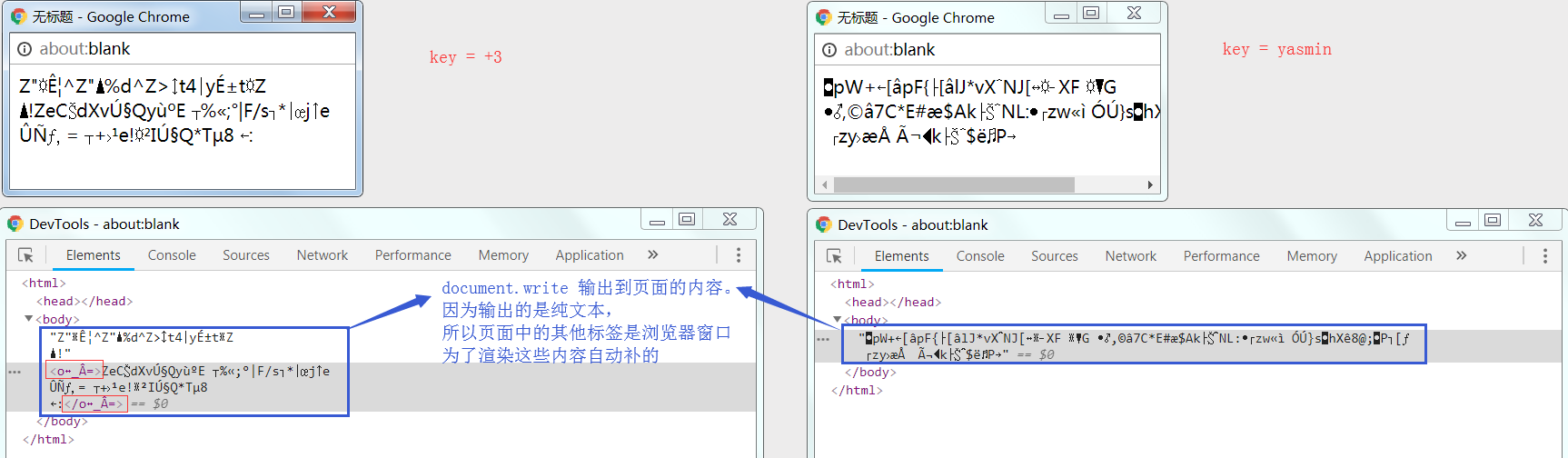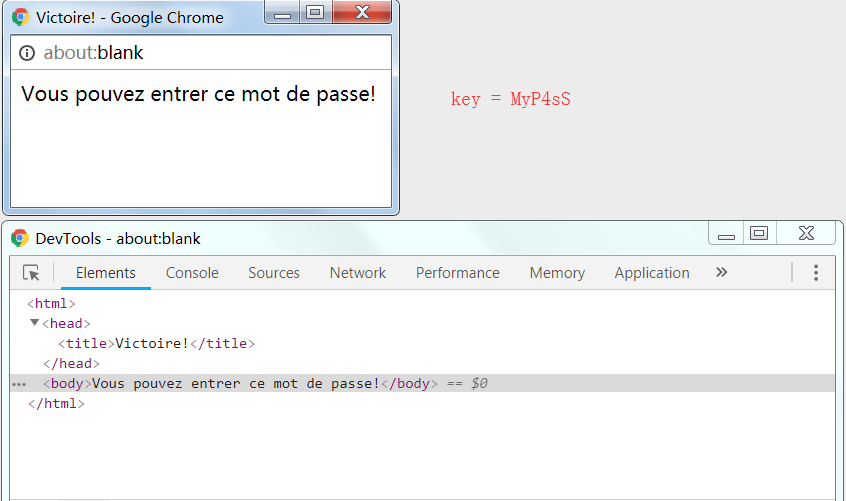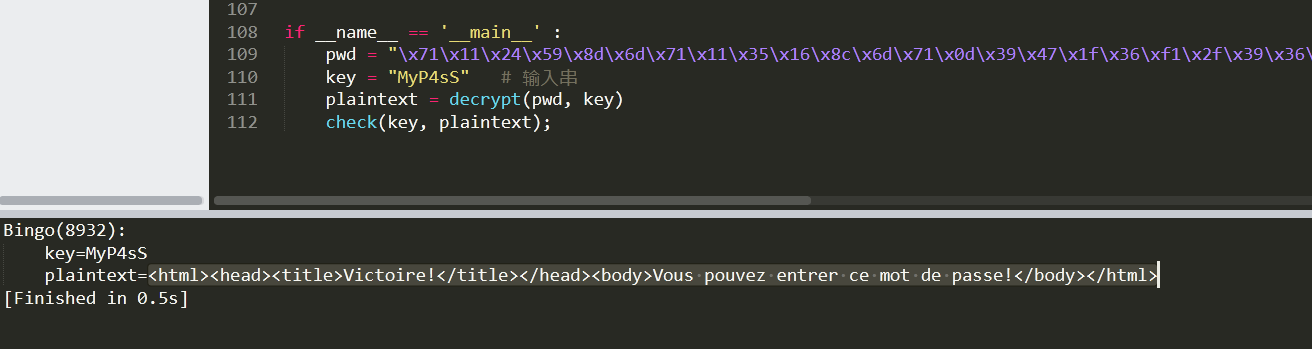# 【Root-Me】 Javascript - Obfuscation 4

## 感慨

• 提示一在题目说明：需要允许弹窗功能
• 提示二在页面源码：凭耐心和直觉

## 源码梳理

``````<html>
<meta http-equiv="Content-Type" content="text/html; charset=UTF-8">
<!--
But du jeu: trouver le bon mdp ! Il vous faudra de la patience et de l intuition!
Si vous avez des problèmes, contactez aaSSfxxx sur #root-me
-->
<script>
var ð = "\x71\x11\x24\x59\x8d\x6d\x71\x11\x35\x16\x8c\x6d\x71\x0d\x39\x47\x1f\x36\xf1\x2f\x39\x36\x8e\x3c\x4b\x39\x35\x12\x87\x7c\xa3\x10\x74\x58\x16\xc7\x71\x56\x68\x51\x2c\x8c\x73\x45\x32\x5b\x8c\x2a\xf1\x2f\x3f\x57\x6e\x04\x3d\x16\x75\x67\x16\x4f\x6d\x1c\x6e\x40\x01\x36\x93\x59\x33\x56\x04\x3e\x7b\x3a\x70\x50\x16\x04\x3d\x18\x73\x37\xac\x24\xe1\x56\x62\x5b\x8c\x2a\xf1\x45\x7f\x86\x07\x3e\x63\x47";
function _(x, y) {
return x ^ y;
}
function __(y) {
var z = 0;
for (var i = 0; i < y; i++) {
z += Math.pow(2, i);
}
return z;
}
function ___(y) {
var z = 0;
for (var i = 8 - y; i < 8; i++) {
z += Math.pow(2, i);
}
return z
}
function ____(x, y) {
y = y % 8;
Ï = __(y);
Ï = (x & Ï) << (8 - y);
return (Ï) + (x >> y);
}
function _____(x, y) {
y = y % 8;
Ï = ___(y);
Ï = (x & Ï) >> (8 - y);
return ((Ï) + (x << y)) & 0x00ff;
}
function ______(x, y) {
return _____(x, y)
}
function _______(_________, key) {
________ = "";
________2 = "";
for (var i = 0; i < _________.length; i++) {
c = _________.charCodeAt(i);
if (i != 0) {
t = ________.charCodeAt(i - 1) % 2;
switch (t) {
case 0:
cr = _(c, key.charCodeAt(i % key.length));
break;
case 1:
cr = ______(c, key.charCodeAt(i % key.length));
break;
}
} else {
cr = _(c, key.charCodeAt(i % key.length));
}
________ += String.fromCharCode(cr);
}
return ________;
}
function __________(þ) {
var ŋ = 0;
for (var i = 0; i < þ.length; i++) {
ŋ += þ["charCodeAt"](i)
}
if (ŋ == 8932) {
var ç = window.open("", "", "\x77\x69\x64\x74\x68\x3d\x33\x30\x30\x2c\x68\x65\x69\x67\x68\x74\x3d\x32\x20\x30");
ç.document.write(þ)
} else {
}
}
__________(_______(ð, prompt("Mot de passe?")));
</script>
<body>
<link rel='stylesheet' property='stylesheet' id='s' type='text/css' href='/template/s.css' media='all'/>
</body>
</html>
``````

``````function xor(x, y) {
return x ^ y;
}

function powsum1(y) {
var sum = 0;
for (var i = 0; i < y; i++) {
sum += Math.pow(2, i);
}
return sum;
}

function powsum2(y) {
var sum = 0;
for (var i = 8 - y; i < 8; i++) {
sum += Math.pow(2, i);
}
return sum;
}

function bit1(x, y) {
y = y % 8;
ps = powsum1(y);
ps = (x & ps) << (8 - y);
return (ps) + (x >> y);
}

function bit2(x, y) {
y = y % 8;
ps = powsum2(y);
ps = (x & ps) >> (8 - y);
return ((ps) + (x << y)) & 0x00ff;
}

function bit(x, y) {
return bit2(x, y)
}

function encrypy(salt, key) {
pwd = "";
unuse = "";
for (var i = 0; i < salt.length; i++) {
c = salt.charCodeAt(i);
if (i != 0) {
switch (pwd.charCodeAt(i - 1) % 2) {
case 0:
n = xor(c, key.charCodeAt(i % key.length));
break;
case 1:
n = bit(c, key.charCodeAt(i % key.length));
break;
}
} else {
n = xor(c, key.charCodeAt(i % key.length));
}
pwd += String.fromCharCode(n);
}
return pwd;
}

function check(pwd) {
var checksum = 0;
for (var i = 0; i < pwd.length; i++) {
checksum += pwd["charCodeAt"](i)
}

if (checksum == 8932) {
var win = window.open("", "", "width=300, height=20");    // 这里对原本的十六进制字符做了解码
win.document.write(pwd)
} else {
alert("Mauvais mot de passe: " + checksum)
}
}

var salt = "\x71\x11\x24\x59\x8d\x6d\x71\x11\x35\x16\x8c\x6d\x71\x0d\x39\x47\x1f\x36\xf1\x2f\x39\x36\x8e\x3c\x4b\x39\x35\x12\x87\x7c\xa3\x10\x74\x58\x16\xc7\x71\x56\x68\x51\x2c\x8c\x73\x45\x32\x5b\x8c\x2a\xf1\x2f\x3f\x57\x6e\x04\x3d\x16\x75\x67\x16\x4f\x6d\x1c\x6e\x40\x01\x36\x93\x59\x33\x56\x04\x3e\x7b\x3a\x70\x50\x16\x04\x3d\x18\x73\x37\xac\x24\xe1\x56\x62\x5b\x8c\x2a\xf1\x45\x7f\x86\x07\x3e\x63\x47"
var key = prompt("Mot de passe?")
var pwd = encrypy(salt, key)
check(pwd);``````

`salt` 的十六进制串因为解码出来还是乱码，所以这里就先保持原样不动。

``````# 异或运算
# 存在逆运算：若 z = xor(x, y) ，则 y = xor(x, z)
def xor(x, y) :
return x ^ y

# 指数求和（除0外结果总是奇数）
# 由于 y 的取值范围只能是 [0, 8), 因此返回值只有 8 种可能：
#   1 => 1
#   2 => 3
#   3 => 7
#   4 => 15
#   5 => 31
#   6 => 63
#   7 => 127
def powsum1(y) :
sum = 0
for i in range(0, y) :
sum += pow(2, i)
return sum

# 指数求和（结果总是偶数）
# 由于 y 的取值范围只能是 [0, 8), 因此返回值只有 8 种可能：
#   0 => 0
#   1 => 128
#   2 => 192
#   3 => 224
#   4 => 240
#   5 => 248
#   6 => 252
#   7 => 254
def powsum2(y) :
sum = 0
for i in range(8 - y, 8) :
sum += pow(2, i)
return sum

# 执行一些位运算（此函数并没有被调用）
# 但因为 powsum1 的缘故，当 x 固定的时候，结果只会是某 8 个固定的 ASCII 码
# 导致输入与输出不是一一映射关系，因此 bit1 不可能存在逆运算
#
# 另外当 x 固定的时候，无论 y 为何值，均有 bit1(x, y) == bit2(x, 8 - y)
def bit1(x, y) :
y = y % 8
ps = powsum1(y)
ps = (x & ps) << (8 - y)
return ps + (x >> y)

# 执行一些位运算
# 但因为 powsum2 的缘故，当 x 固定的时候，结果只会是某 8 个固定的 ASCII 码
# 导致输入与输出不是一一映射关系，因此 bit2 不可能存在逆运算
#
# 另外当 x 固定的时候，无论 y 为何值，均有 bit2(x, y) == bit1(x, 8 - y)
def bit2(x, y) :
y = y % 8
ps = powsum2(y)
ps = (x & ps) >> (8 - y)
return (ps + (x << y)) & 0x00ff

# 执行一些位运算
def bit(x, y) :
return bit2(x, y)   # bit1(x, y) == bit2(x, 8 - y)

# 使用输入串 key 对盐 salt 循环加密，得到加密串 pwd
def encrypt(salt, key) :
pwd = ''
key_len = len(key)
if key_len > 0 :
for i, c in enumerate(salt) :
x = ord(c)
y = ord(key[i % key_len])

if i > 0 and ord(pwd[i - 1]) % 2 != 0 :
y = y % 8
z = bit(x, y)
else :
z = xor(x, y)

pwd += chr(z)
return pwd

# 检查加密串 pwd 的校验和
# 当校验和为 8932 时，把 pwd 的内容输出到弹出窗口
def check(key, pwd) :
checksum = 0
for c in pwd :
checksum += ord(c)   # 校验和

flag = (checksum == 8932)
if flag :
# 原 js 代码：
# var win = window.open("", "", "width=300, height=20")
# win.document.write(pwd)
pass

print('%s(%i): \n\tkey=%s\n\tpwd=%s' % ('Bingo' if flag else 'Fail', checksum, key, pwd))
return flag

if __name__ == '__main__' :
salt = "\x71\x11\x24\x59\x8d\x6d\x71\x11\x35\x16\x8c\x6d\x71\x0d\x39\x47\x1f\x36\xf1\x2f\x39\x36\x8e\x3c\x4b\x39\x35\x12\x87\x7c\xa3\x10\x74\x58\x16\xc7\x71\x56\x68\x51\x2c\x8c\x73\x45\x32\x5b\x8c\x2a\xf1\x2f\x3f\x57\x6e\x04\x3d\x16\x75\x67\x16\x4f\x6d\x1c\x6e\x40\x01\x36\x93\x59\x33\x56\x04\x3e\x7b\x3a\x70\x50\x16\x04\x3d\x18\x73\x37\xac\x24\xe1\x56\x62\x5b\x8c\x2a\xf1\x45\x7f\x86\x07\x3e\x63\x47";
key = ""   # 输入串
pwd = encrypt(salt, key)
check(key, pwd);``````

_(x, y) xor(x, y) xor(x, y) 异或运算（存在逆运算

__(y) powsum1(y) powsum1(y) 指数求和（被 bit1 调用）
y 取值范围为 [0, 8)]，对应输出固定为
0, 1, 3, 7, 15, 31, 63, 127
___(y) powsum2(y) powsum2(y) 指数求和（被 bit2 调用）
y 取值范围为 [0, 8)]，对应输出固定为
0, 128, 192, 224, 240, 248, 252, 254
____(x, y) bit1(x, y) bit1(x, y) 执行一些位运算（此函数并没有被调用）

_____(x, y) bit2(x, y) bit2(x, y) 执行一些位运算（不存在逆运算

______(x, y) bit(x, y) bit(x, y) 无任何作用

_______(ð, key) encrypt(salt, key) encrypt(salt, key) 使用输入串 key 对盐 salt 循环加密

__________(þ) check(pwd) check(key, pwd) 检查加密串 pwd 每个字符的 ASCII 码之和

ð salt salt 盐（固定值，十六进制串）
key key key 输入字符串（本题需要推导的最终 flag
þ pwd pwd salt 与 key 加密得到的字符串

## 背景分析

• 加密算法的逻辑
• `salt`
• 密码 `pwd` 的校验和

## 密码爆破

• 以 ASCII 表作为 `key` 的每个字符范围进行枚举，令 `key` 的长度依次为 1 个字符、2个字符、3个字符….
• 枚举从 darkweb 统计出来的 Top 10000 的弱密码作为 `key`（密码表可在 SecList 下载）

`key` 的每个字符一直在通过 取模运算，循环 地对 `salt` 的每个字符依次执行某个操作（`xor``bit`

`+3``+3+3``+3+3+3``yasmin``yasminyasmin``yasminyasminyasmin`、……

## 进一步分析

• `pwd` => `plaintext`
• `salt` => `pwd`
• `encrypt(salt, key)` => `decrypt(pwd, key)`
``````# 使用输入串 key 对密文 pwd 循环解密，得到明文 plaintext
def decrypt(pwd, key) :
plaintext = ''
key_len = len(key)
if key_len > 0 :
for i, c in enumerate(pwd) :
x = ord(c)
y = ord(key[i % key_len])

if i > 0 and ord(plaintext[i - 1]) % 2 != 0 :
y = y % 8
z = bit(x, y)
else :
z = xor(x, y)

plaintext += chr(z)
return plaintext

# 检查明文 plaintext 的校验和
# 当校验和为 8932 时，把 plaintext 的内容输出到弹出窗口
def check(key, plaintext) :
checksum = 0
for c in plaintext :
checksum += ord(c)   # 校验和

flag = (checksum == 8932)
if flag :
# 原 js 代码：
# var win = window.open("", "", "width=300, height=20")
# win.document.write(pwd)
pass

print('%s(%i): \n\tkey=%s\n\tplaintext=%s' % ('Bingo' if flag else 'Fail', checksum, key, plaintext))
return flag

if __name__ == '__main__' :
pwd = "\x71\x11\x24\x59\x8d\x6d\x71\x11\x35\x16\x8c\x6d\x71\x0d\x39\x47\x1f\x36\xf1\x2f\x39\x36\x8e\x3c\x4b\x39\x35\x12\x87\x7c\xa3\x10\x74\x58\x16\xc7\x71\x56\x68\x51\x2c\x8c\x73\x45\x32\x5b\x8c\x2a\xf1\x2f\x3f\x57\x6e\x04\x3d\x16\x75\x67\x16\x4f\x6d\x1c\x6e\x40\x01\x36\x93\x59\x33\x56\x04\x3e\x7b\x3a\x70\x50\x16\x04\x3d\x18\x73\x37\xac\x24\xe1\x56\x62\x5b\x8c\x2a\xf1\x45\x7f\x86\x07\x3e\x63\x47";
key = ""   # 输入串
plaintext = decrypt(pwd, key)
check(key, plaintext);``````

## 调整思路

**有一密文 `pwd` ， 需要找到其加密密钥 `key` 解密成明文 `plaintext`，若解密成功则把 `plaintext` 输出到一个弹窗**。

## 为什么是弹窗

`NB : You will have to enable popups in order to solve this challenge!`

``````if (checksum == 8932) {
var win = window.open("", "", "width=300, height=20");
win.document.write(pwd)
} else {
alert("Mauvais mot de passe: " + checksum)
} ``````

○ URL：可选，声明了要在新窗口中显示的页面的 URL。

如果省略了这个参数，或者它的值是空字符串，那么新窗口就不会显示任何页面内容

○ name：可选，声明了新窗口的名称

○ features：可选，声明了新窗口要显示的标准浏览器的特征

○ replace：可选，本题代码中并没有使用这个参数• 弹窗显示了意义不明的乱码内容，而且为了显示乱码内容，弹窗自动添加了 <html> 等标签（因为使用 python 运行时，并没有输出这些标签，而且 `window.open` 的 URL 为空，即页面本身不会有任何内容，所以我肯定这些标签是弹窗自动加的，与 `window.open``document.write` 无关）
• 不同的 `key` 值得到输出到弹窗的内容不同（意味着很可能有一个 `key` 会使得内容变成明文）
• `key` 值为 `+3` 时，不难发现乱码中有一对闭合标签 `<o_Â=>`

## 逆推

`decrypt` 逻辑可知：

`plaintext = xor( pwd, key )` ，现已知 `pwd = '\x71'``plaintext = '<'`

pwd[i] \x71 \x11 \x24 \x59 \x8d \x6d \x71 ……
plaintext[i-1] - ……
plaintext[i] < h t m l > < ……

key[i] M y P 4 ?（未知） S M ……

## Bingo

`plaintext = bit( pwd, key ) = 'l'` ，但是从算术上无法逆推 `key` 值。

（其实 `?` 不用爆破也能猜到不是 `s` 就是 `S`，因为很明显 `MyP4sS` 就是 `MyPASS` 的变体）`<html><head><title>Victoire!</title></head><body>Vous pouvez entrer ce mot de passe!</body></html>`## 答案下载

flag 下载后的 flagzip 的文件需要手动更改后缀为 `*.zip`，然后解压即可（为了避免直接刷答案）

目录